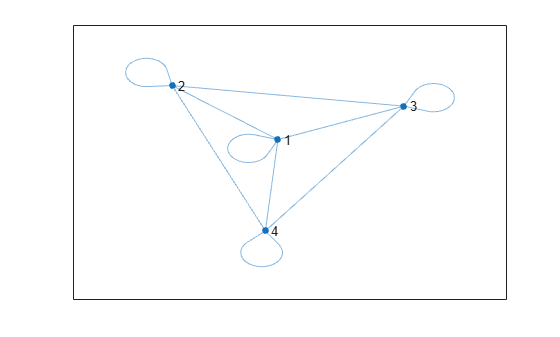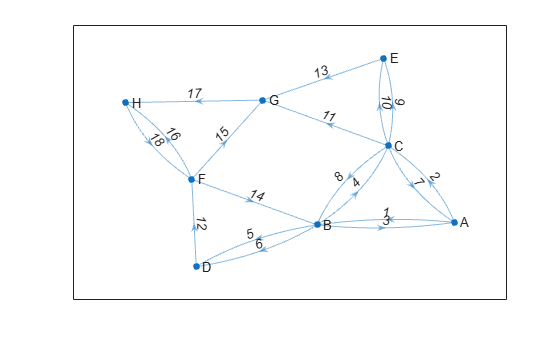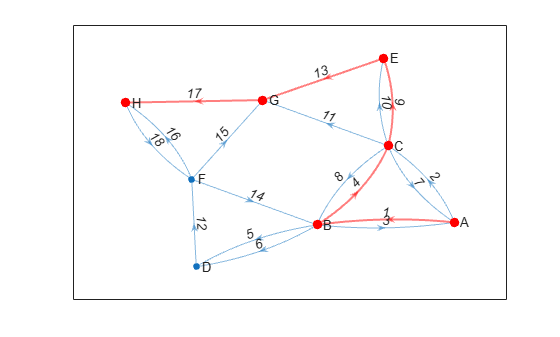# allpaths

Find all paths between two graph nodes

## Syntax

``paths = allpaths(G,s,t)``
``[paths,edgepaths] = allpaths(G,s,t)``
``[___] = allpaths(G,s,t,Name,Value)``

## Description

example

````paths = allpaths(G,s,t)` returns all paths in graph `G` that start at source node `s` and end at target node `t`. The output `paths` is a cell array where the contents of each cell `paths{k}` lists nodes that lie on a path.```

example

````[paths,edgepaths] = allpaths(G,s,t)` also returns the edges on each path from `s` to `t`. The output `edgepaths` is a cell array where `edgepaths{k}` gives the edges along the corresponding path, `paths{k}`.```

example

````[___] = allpaths(G,s,t,Name,Value)` specifies additional options using one or more name-value arguments. You can use any of the output argument combinations in previous syntaxes. For example, you can specify `MaxNumPaths` and a scalar to limit the number of paths returned.```

## Examples

collapse all

Create an adjacency matrix for a complete graph with four nodes, and then create an undirected graph from the adjacency matrix. Plot the graph.

```A = ones(4); G = graph(A); plot(G)```Calculate all paths in the graph that begin at node 1 and end at node 3.

`paths = allpaths(G,1,3)`
```paths=5×1 cell array {[ 1 2 3]} {[1 2 4 3]} {[ 1 3]} {[1 4 2 3]} {[ 1 4 3]} ```

The second output argument of `allpaths` returns the edges that are along each path. This is particularly useful for multigraphs, where the edge index is required to uniquely identify the edges on the path.

Create a directed multigraph with eight nodes and 18 edges. Specify names for the nodes. Plot the graph with labeled nodes and edges.

```s = [1 1 2 2 3 3 2 2 4 6 8 6 6 7 3 3 5 3]; t = [2 3 1 3 2 1 4 4 6 2 6 7 8 8 5 5 7 7]; names = {'A','B','C','D','E','F','G','H'}; G = digraph(s,t,[],names); p = plot(G,'EdgeLabel',1:numedges(G));```Calculate all paths between node A and node H. Specify two output arguments to also return the edge indices for edges along each path.

`[paths,edgepaths] = allpaths(G,'A','H');`

View the nodes and edges along the first path.

`paths{1}`
```ans = 1x6 cell {'A'} {'B'} {'C'} {'E'} {'G'} {'H'} ```
`edgepaths{1}`
```ans = 1×5 1 4 9 13 17 ```

Highlight the nodes and edges along the first path.

`highlight(p,'Edges',edgepaths{1},'EdgeColor','r','LineWidth',1.5,'NodeColor','r','MarkerSize',6)`Use the `'MaxNumPaths'`, `'MaxPathLength'`, and `'MinPathLength'` options to limit the number of paths returned by `allpaths`.

Create an adjacency matrix for a complete graph with 20 nodes. Create an undirected graph from the adjacency matrix, omitting self-loops.

```A = ones(20); G = graph(A,'omitselfloops');```

Since all of the nodes in the graph are connected to all other nodes, there are a large number of paths in the graph between any two nodes (more than `1.7e16`). Therefore, it is not feasible to calculate all of the paths between two nodes since the results will not fit in memory. Instead, calculate the first 10 paths from node 2 to node 5.

`paths1 = allpaths(G,2,5,'MaxNumPaths',10)`
```paths1=10×1 cell array {[ 2 1 3 4 5]} {[ 2 1 3 4 6 5]} {[ 2 1 3 4 6 7 5]} {[ 2 1 3 4 6 7 8 5]} {[ 2 1 3 4 6 7 8 9 5]} {[ 2 1 3 4 6 7 8 9 10 5]} {[ 2 1 3 4 6 7 8 9 10 11 5]} {[ 2 1 3 4 6 7 8 9 10 11 12 5]} {[ 2 1 3 4 6 7 8 9 10 11 12 13 5]} {[2 1 3 4 6 7 8 9 10 11 12 13 14 5]} ```

Now calculate the first 10 paths between node 2 and node 5 that have a path length less than or equal to 2.

`paths2 = allpaths(G,2,5,'MaxNumPaths',10,'MaxPathLength',2)`
```paths2=10×1 cell array {[ 2 1 5]} {[ 2 3 5]} {[ 2 4 5]} {[ 2 5]} {[ 2 6 5]} {[ 2 7 5]} {[ 2 8 5]} {[ 2 9 5]} {[2 10 5]} {[2 11 5]} ```

Finally, calculate the first 10 paths between node 2 and node 5 that have a path length greater than or equal to 3.

`paths3 = allpaths(G,2,5,'MaxNumPaths',10,'MinPathLength',3)`
```paths3=10×1 cell array {[ 2 1 3 4 5]} {[ 2 1 3 4 6 5]} {[ 2 1 3 4 6 7 5]} {[ 2 1 3 4 6 7 8 5]} {[ 2 1 3 4 6 7 8 9 5]} {[ 2 1 3 4 6 7 8 9 10 5]} {[ 2 1 3 4 6 7 8 9 10 11 5]} {[ 2 1 3 4 6 7 8 9 10 11 12 5]} {[ 2 1 3 4 6 7 8 9 10 11 12 13 5]} {[2 1 3 4 6 7 8 9 10 11 12 13 14 5]} ```

## Input Arguments

collapse all

Input graph, specified as either a `graph` or `digraph` object. Use `graph` to create an undirected graph or `digraph` to create a directed graph.

Example: `G = graph(1,2)`

Example: `G = digraph([1 2],[2 3])`

Source and target node IDs, specified as separate arguments of node indices or node names.

ValueExample
Scalar node index`1`
Character vector node name`'A'`
String scalar node name`"A"`

Example: `allpaths(G,2,5)` computes all paths between node 2 and node 5.

Example: `allpaths(G,'node1','node2')` computes all paths between the named nodes `node1` and `node2`.

### Name-Value Arguments

Specify optional comma-separated pairs of `Name,Value` arguments. `Name` is the argument name and `Value` is the corresponding value. `Name` must appear inside quotes. You can specify several name and value pair arguments in any order as `Name1,Value1,...,NameN,ValueN`.

Example: `allpaths(G,s,t,'MaxNumPaths',100)` returns only the first 100 results in the lexicographic ordering of paths.

Maximum number of paths, specified as the comma-separated pair consisting of `'MaxNumPaths'` and a nonnegative integer scalar. This option is useful when the number of paths between two nodes grows large enough to hit memory limits. You can specify `MaxNumPaths` to limit the number of paths returned by `allpaths` so that the results fit within available memory.

Example: `allpaths(G,s,t,'MaxNumPaths',100)`

Maximum path length, specified as the comma-separated pair consisting of `'MaxPathLength'` and a nonnegative integer scalar. This option filters the results returned by `allpaths` so that no paths with length larger than the specified limit are returned. The length of a path is measured by the number of edges in it, ignoring edge weights.

To find paths with a range of lengths, specify both `'MaxPathLength'` and `'MinPathLength'`. To find paths with an exact specified length, specify the same value for both `'MaxPathLength'` and `'MinPathLength'`.

Example: `allpaths(G,s,t,'MaxPathLength',4)` returns paths that have a length less than or equal to 4.

Example: `allpaths(G,s,t,'MinPathLength',3,'MaxPathLength',5)` returns paths that have a length of 3, 4, or 5.

Minimum path length, specified as the comma-separated pair consisting of `'MinPathLength'` and a nonnegative integer scalar. This option filters the results returned by `allpaths` so that no paths with length smaller than the specified limit are returned. The length of a path is measured by the number of edges in it, ignoring edge weights.

To find paths with a range of lengths, specify both `'MaxPathLength'` and `'MinPathLength'`. To find paths with an exact specified length, specify the same value for both `'MaxPathLength'` and `'MinPathLength'`.

Example: `allpaths(G,s,t,'MinPathLength',2)` returns paths that have a length greater than or equal to 2.

Example: `allpaths(G,s,t,'MinPathLength',3,'MaxPathLength',5)` returns paths that have a length of 3, 4, or 5.

## Output Arguments

collapse all

Paths between specified nodes, returned as a cell array. Each element `paths{k}` contains the nodes that lie along one of the paths between the specified source and target nodes. The paths are returned in lexicographical order. If the source and target nodes `s` and `t` specify the same node, then by convention `allpaths` returns a single path containing that node. If node `t` is unreachable from node `s`, then `paths` is empty.

The data type of the entries in `paths` depends on the way `s` and `t` are specified:

• If `s` and `t` are specified as numeric node indices, then each element `paths{k}` is a numeric vector of node indices.

• If `s` and `t` are specified as string node names, then each element `paths{k}` is a string array of node names.

• If `s` and `t` are specified as character vector node names, then each element `paths{k}` is a cell array of character vector node names.

Edges along each path, returned as a cell array. Each element `edgepaths{k}` contains the edge indices for edges that lie along the corresponding path, `paths{k}`. If node `t` is unreachable from node `s`, then `edgepaths` is empty.

## Tips

• The number of paths in a graph depends heavily on the structure of the graph. For some graph structures, the number of paths can grow exponentially with the number of nodes. For example, a complete graph with 12 nodes given by `G = graph(ones(12))` contains nearly 10 million paths between any two of its nodes. Use the `MaxNumPaths`, `MaxPathLength`, and `MinPathLength` name-value pairs to control the output of `allpaths` in these cases.